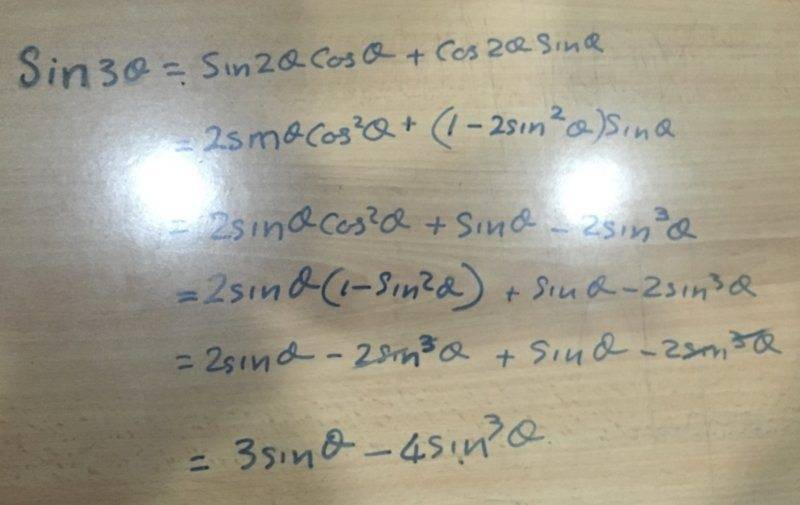# I need help with this identity

## Homework Statement

8 sin3 θ – 6 sin θ + 1 = 0

The answer includes changing this to
-2sin3θ+1=0

## Homework Equations

The double angle identities
Sin2θ=sinθcosθ+cosθsinθ

## The Attempt at a Solution

I do not know how to get started with this question

PeroK
Homework Helper
Gold Member
2020 Award

## Homework Statement

8 sin3 θ – 6 sin θ + 1 = 0

The answer includes changing this to
-2sin3θ+1=0

## Homework Equations

The double angle identities
Sin2θ=sinθcosθ+cosθsinθ

## The Attempt at a Solution

I do not know how to get started with this question

You could try looking at ##\sin(3\theta) = \sin(2\theta + \theta)##.

•mattt
You could try looking at ##\sin(3\theta) = \sin(2\theta + \theta)##.
I am familiar with that
Which is the double angle identity
But I fail to see the the relation
I believe that I am missing the transition identity which brings them together
Could you point it out for me please

PeroK
Homework Helper
Gold Member
2020 Award
I am familiar with that
Which is the double angle identity
But I fail to see the the relation
I believe that I am missing the transition identity which brings them together
Could you point it out for me please

Expand the expression in post #2. And then have another think.

Last edited:
Expand the expression in post #2. And then have another think.
This what I came to when I expanded
What do I do from there#### Attachments

Ray Vickson
Homework Helper
Dearly Missed
This what I came to when I expanded
What do I do from there
View attachment 221431

So, what do you get for ##2 \sin 3\theta ?##

So, what do you get for ##2 \sin 3\theta ?##
Oh
You mean twice that huh ok let me see

## Homework Statement

8 sin3 θ – 6 sin θ + 1 = 0

The answer includes changing this to
-2sin3θ+1=0

## Homework Equations

The double angle identities
Sin2θ=sinθcosθ+cosθsinθ

## The Attempt at a Solution

I do not know how to get started with this question
It can't be an identity, because when I substitute 90 degrees for theta it comes out to - 8 - 6 + 1 = 0. What is it you are supposed to do? Solve for theta?

Mark44
Mentor
You could try looking at ##\sin(3\theta) = \sin(2\theta + \theta)##.

I am familiar with that
Which is the double angle identity
No, PeroK is setting up to use an addition formula, not a double angle identity.

You seem to be confused about the concepts of proving an identity versus solving a conditional equation. This problem, which you mistakenly titled "I need help with this identity," actually entails solving an equation.

The difference between these two kinds of equations is that an identity is true for all values of the variable; for example, ##\sin^2(\theta) + \cos^2(\theta) = 1##. A conditional equation is one that is true for a limited number of values of the variable; for example ##x^2 - 2x + 1 = 0##.

TeethWhitener